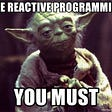# The First Few Lines of Arrays in Rust

A fixed-size array, denoted [T; N], for the element type, T, and the non-negative compile-time constant size, N.

This is what you find when you read the first few lines of the documentation about the array type in Rust.

Let’s go through this piece by piece.
To begin with we have the following

A fixed-size array …

Alright, so now we know that the array will never grow or shrink in memory. If you create an array with the capacity to hold 5 elements of the type bool it will forever be an array that can hold 5 bools.

We continue and find the description

… denoted [T; N]

The [T; N] tells us the format to use when declaring an array.
But was is T and N?

… for the element type, T

The T refers to the type of the arrays elements. If we wanted to store numbers in the array the T could refer to the i32 type in Rust, [i32; N].
To better understand N we need read the next part which is

… and the non-negative compile-time constant size, N.

The N represents the size of our array, and the documentation mentions two rules that N has to follow.

The first rule
N needs to be a non-negative number.
Let’s disobey that rule and see what happens :-)

`let array_with_negative_size: [i32; -3];`

This produces the compiler error

`error[E0600]: cannot apply unary operator `-` to type `usize``

With the error in hand we can see that the compiler is expecting usize as the type of N. The type usize represents an unsigned integer, so it can never be less than 0.

The second rule
N needs to be a compile time constant. If what ever expression used for N is not replaceable with a constant value, the rule is broken.

Here we try using the parameter n passed to the function as the value for N.

`fn compile_time_array_size(n: usize) {  let array_with_positive_size: [i32; n];}`

This produces the compiler error

`error[E0435]: attempt to use a non-constant value in a constant`

And there we have it.

# Going further …

There are two syntactic forms for creating an array:

A list with each element, i.e., [x, y, z].

A repeat expression [x; N], which produces an array with N copies of x. The type of x must be Copy.

This is what you find if you keep reading the documentation.
It highlights the two ways to actually create an array.

## Method one

A list with each element, i.e., [x, y, z].

`let array_of_numbers = [0, 1, 1, 2, 3, 5, 8];`

What did we declare in this case?

To begin with, the compiler can figure out that we have 7 elements in our array and will use that to set the size(N) of the array.

Rust defaults to i32 for numbers, which means that we’ve just declared an array with 7 elements of i32s, [i32; 7].

What happens if you have a literal where any of the elements values exceed the capabilities of an i32?

`let array_of_numbers = [0, 1, 10_000_000_000];`

The compiler will complain with

`error: literal out of range for `i32``

From this we can tell that Rust won’t help us change the type automatically to something more appropriate, like an i64. For this we need to do the following

`let array_of_numbers: [i64; 3] = [0, 1, 10_000_000_000];`

We now have an array that contains 3 i64 elements.

The specific values in the array literal can be described with more than constant values. You could, for example, set the values with an expression.

`fn array_littera(s: String) {  let array = [s.len(), s.len() + 1, s.len() + 2];}`

## Method two

A repeat expression [x; N], which produces an array with N copies of x. The type of x must be Copy.

Keeping our attention on arrays, we will just glance over the “must be Copy” part and just understand that — the Copy trait is simply the thing that allows us to copy values. The more important part is to understand what the copy-functionality is used for.

The N still represents the size of the array but we now have x, which is a “repeat expression”. This will in other words take x and use it as the initial value for all of our elements. Now we see why it needs to be copied, the value we give x needs to repeatedly copied into the array.

The documentation uses the word “expression”, we can do a lot with an expression in Rust. This means that we can be a lot more dynamic in how we create our x value.

Let’s play around with this a bit :-)

`fn create_array_with_fn_as_repeatable_expression(string: String) {  let array = [string.len(); 2];}`

Or … you could do this (you probably shouldn’t).

`fn create_array_with_repeatable_expression(input: u128) {  let array_of_number = [{    let modulus = 10 * input;    std::time::SystemTime::now()      .duration_since(std::time::UNIX_EPOCH)      .map(|d| d.as_millis())      .map(|n| n % modulus)      .unwrap_or(10)   }; 3];}`

But why?
Why are these two methods good to know?
One reason is that you can’t work with an array if it’s uninitialized.

This will, for example, fail

`let array: [i32; 10];let length = array.len();`

and produces the compiler error

`error[E0381]: borrow of possibly-uninitialized variable: `array``

So these two methods allow you to initialize an array by either

• explicitly setting each value
• or by supplying a value to be copied into each element of the array

The end.

--

--

--

## More from Analytics Vidhya

Analytics Vidhya is a community of Analytics and Data Science professionals. We are building the next-gen data science ecosystem https://www.analyticsvidhya.com## robert barlin

… a little bit of everything :)

## Using Binary Mode in Haskell